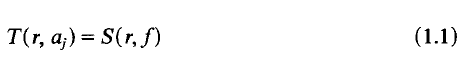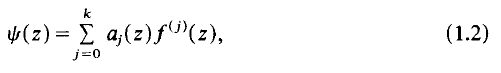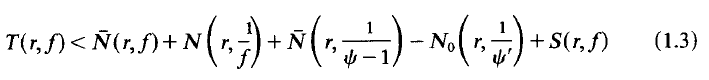Skip to main content Accessibility help
Home

# On Hayman's alternative

## Extract

Suppose that f(z) is non-constant and meromorphic in the plane and that, for some k≥= 1, a0(z),…, ak(z) are meromorphic in the plane withfor j' = 0,…, k. Here, using standard notation from , S(r,f) denotes any quantity satisfying S(r,f) = o(T(r,f)) as r→ ∞, possibly outside a set of finite linear measure. Then, settingwe have ([3, p. 57])

Theorem A. Suppose that f(z) is non-constant and meromorphic in the plane, and thatψ (z) given by (1.2) and (1.1) and is non-constant. Thenwhere N0(r, l/ψ') counts only zeros of ψ' which are not zeros of ψ − 1, and thecounting functions count points without regard to multiplicity.

## References

Hide All
MathJax
MathJax is a JavaScript display engine for mathematics. For more information see http://www.mathjax.org.

## Metrics

### Full text viewsFull text views reflects the number of PDF downloads, PDFs sent to Google Drive, Dropbox and Kindle and HTML full text views.

Total number of HTML views: 0
Total number of PDF views: 0 *Loading metrics...

### Abstract viewsAbstract views reflect the number of visits to the article landing page.

Total abstract views: 0 *Loading metrics...

* Views captured on Cambridge Core between <date>. This data will be updated every 24 hours.

Usage data cannot currently be displayed